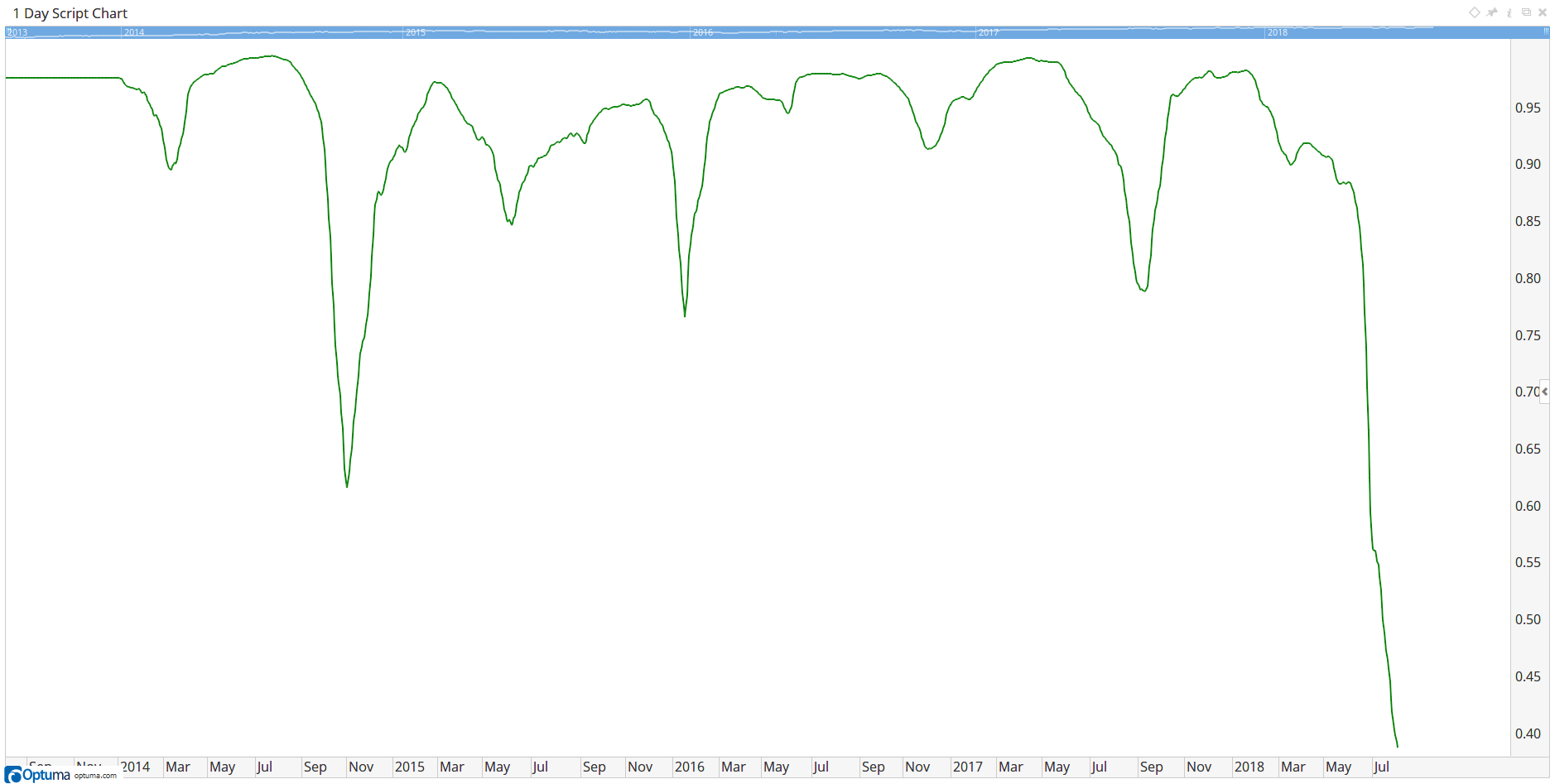Select Page

# Reply To: Plotting average of three correlation coefficients

Optuma Forums Optuma Scripting Plotting average of three correlation coefficients Reply To: Plotting average of three correlation coefficients

#47779

Hi Prashant,

Your best option is to open a Script Chart for NIFTY500 and then use this formula to plot the average 100 day correlation:

The current value is 0.39, which is the average of the tools when plotted on the chart: